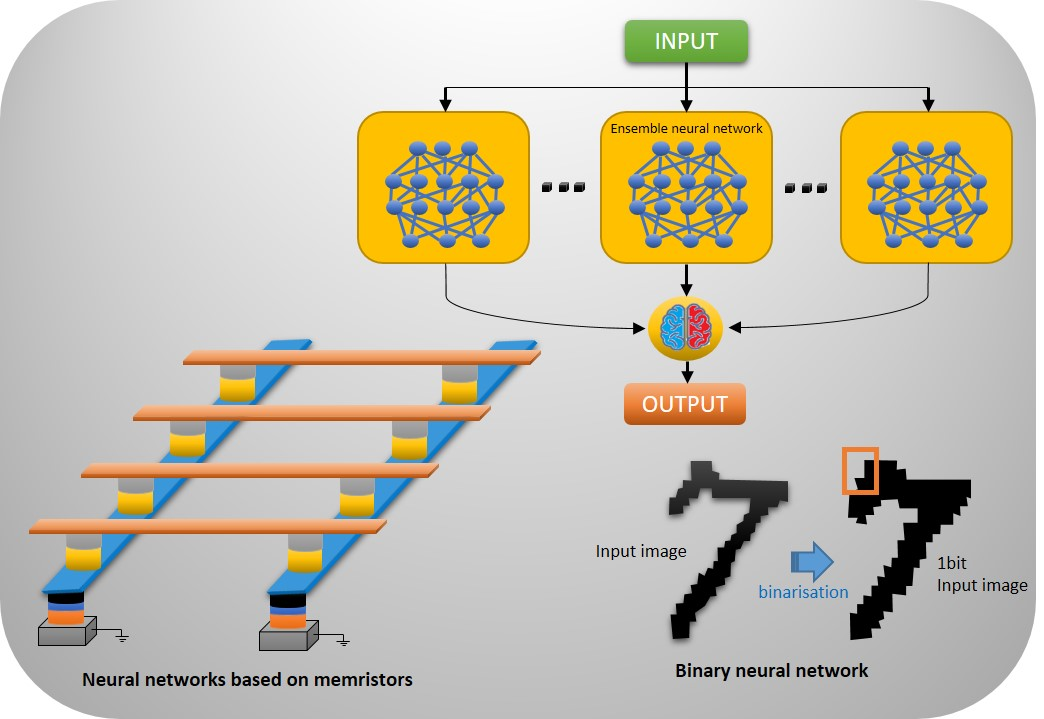# Spintronic memristor based neural networkConvolutional neural networks are adapted to perform cognitive tasks such as image and video recognition, or language processing. In order to implement these networks more efficiently, researchers at IRIG have integrated spintronic devices in an architecture combining a Binary Ensemble Convolutional Neural Network. Specific tools allowed to evaluate this disruptive combination that is less complex and consumes less power.

Published on 6 March 2023

Convolutional neural networks (CNN) are efficient for certain tasks such as image or text recognition. However, the classical design of a computer in which the processor and the memory are physically separated is not compatible with a neural network. Indeed, the calculations requiring that the synapses exchange frequently between the neurons the information stored in the memory (we speak of synaptic weight) that causes a considerable slowdown. Researchers at IRIG have therefore used spintronic devices that allow a parallel implementation of the networks in which the calculation and storage are integrated in the same block.

Researchers have developed electronic memories called memristors whose resistance changes continuously as a function of applied current. In a memory architecture called "crossbar", the memristors code the synaptic weight as an electrical resistance, in order to perform calculations with analog currents that will be converted into digital values. The goal is to realize a convolutional neural network based on memristors, which keeps the same performances while minimizing the complexity and without additional hardware cost. However, as the manufacturing process of these spintronic devices is recent, the accuracy of the computation still needs to be made reliable. Moreover, the synaptic weights can only take two binary states which makes it difficult to access different resistance levels in order to mimic a synapse.

The researchers tested an architecture using two concepts to mitigate these issues. The first one is an ensemble network, according to the "wisdom of crowds" concept, where the global network is replaced by several smaller, less accurate but much simpler networks, which are trained with different samples extracted from the same data set. The results obtained by these networks are then compared to obtain a decision, the accuracy of which is comparable with that of a single network.
The second concept is a binary type network in which the synaptic weights take only two states, contrary to the classical network whose weights vary continuously. Thanks to these two concepts, spintronic devices allow calculations with almost no loss of precision.Figure: neural networks based on spintronic devices (left) combinated with Ensemble neural netwok (up) and Binary neural network (right).

This solution was evaluated on different datasets for image recognition. The architecture of the ensemble network allowed an optimization of the hardware cost, as the number of neurons is reduced by 92% and the number of synapses by 95%, and this with an accuracy similar to that of a single equivalent convolutional network. In addition, a 95% reduction in the number of clock cycles and 97% reduction in the number of memory accesses was observed. And finally, the use of spin-orbit coupled devices allows for a further three orders of magnitude reduction in power consumption due to reduced computational currents.
Convolutional Neural network (CNN) is a class of artificial neural network most commonly applied to analyze visual imagery.
Memristor is a non-linear two-terminal electrical component relating electric charge and magnetic flux linkage.

Top page